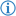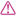The divide operator is an arithmetic operator which divides a number object by another.

Its token is named DIVISION.

# Phrases

## InfixInfix notation can be achieved using the phrase `divided by` or `over`.

Its FDS is

### Example

`I wrote`` ``the number`` ``8`` ``divided by`` ``the number`` ``2``.`

This prints `4`.

Its Java equivalent is as follows:

```System.out.println((double)8 / (double)2);
```

## PrefixPrefix notation can be achieved using the phrase `divide` and the infix separator `and` or `by`.

Its FDS is

### Example

`I wrote`` ``divide`` ``the number`` ``8`` ``by`` ``the number`` ``2``.`

This has the same effect as the above example.